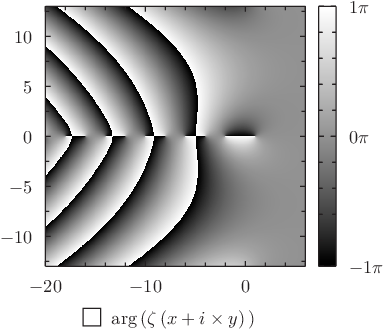# Pyxplot

## Examples - Riemann zetaDownload this example:

An example of the color map plotting style

Pyxplot's colormap plot style produces a color representation of how a function varies across the x-y plane, where different colors represent different values of the function. Here a grayscale color map is produced of the argument of the Riemann zeta function; several branch cuts are clearly visible.

### Script

set numerics complex
set sample grid 800x800
set nogrid
set size square
set key below

set c1range[-pi:pi]
set c1format r"$%s\pi$"%(c/pi)

plot [-20:6][-13:13] arg(zeta(x+i*y)) with colormap